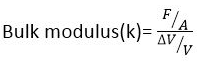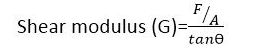Mechanics

# Difference between young’s modulus, bulk modulus and shear modulus

The basic difference between young’s modulus, bulk modulus, and shear modulus is that Young’s modulus is the ratio of tensile stress to tensile strain, the bulk modulus is the ratio of volumetric stress to volumetric strain and shear modulus is the ratio of shear stress to shear strain.

## Young’s modulus

The ratio of tensile stress to tensile strain is called young’s modulus.

## Bulk modulus

For three-dimensional deformation, when the volume is involved, then the ratio of applied stress to volumetric strain is called Bulk modulus. Mathematically it is expressed as:

### Bulk modulus formulaWhere ΔV is the change in original volume V.

## Shear modulus

The ratio of shear stress and shear strain is called shear modulus. Mathematically it is expressed as:

### Shear modulus formulaElastic constants for some of the materials are given in the table:

 Material Young’s modulus 109Nm-2 Bulk modulus 109Nm-2 Shear modulus 109Nm-2 Aluminum 70 70 30 Bone 15 – 80 Copper 110 140 44 Diamond 1120 540 450 Glass 55 31 23 Steel 200 160 84 Tungsten 390 200 150 Water 0 2.2 0 Lead 15 7.7 5.6